Скачать презентацию MUSSELBURGH GRAMMAR SCHOOL APPLIED ELECTRONICS Outcome 2 Gary

578f68bb24c7bf7e73e790038f1a3fb2.ppt

• Количество слайдов: 67MUSSELBURGH GRAMMAR SCHOOL APPLIED ELECTRONICS Outcome 2 Gary Plimer 2004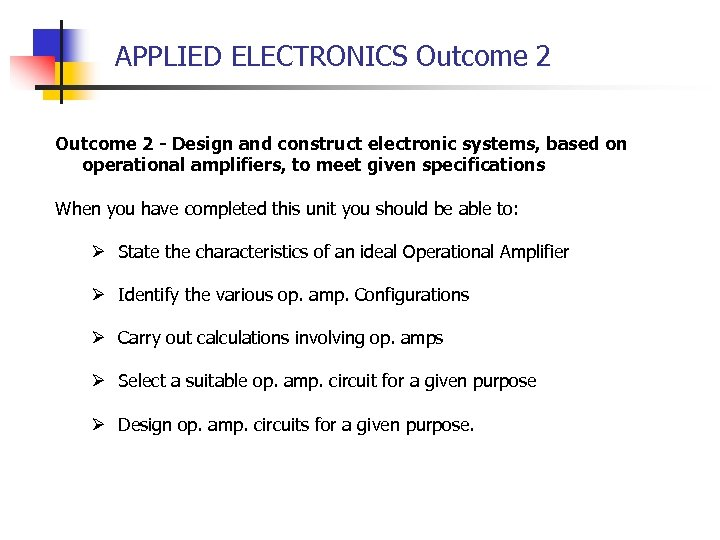APPLIED ELECTRONICS Outcome 2 - Design and construct electronic systems, based on operational amplifiers, to meet given specifications When you have completed this unit you should be able to: Ø State the characteristics of an ideal Operational Amplifier Ø Identify the various op. amp. Configurations Ø Carry out calculations involving op. amps Ø Select a suitable op. amp. circuit for a given purpose Ø Design op. amp. circuits for a given purpose.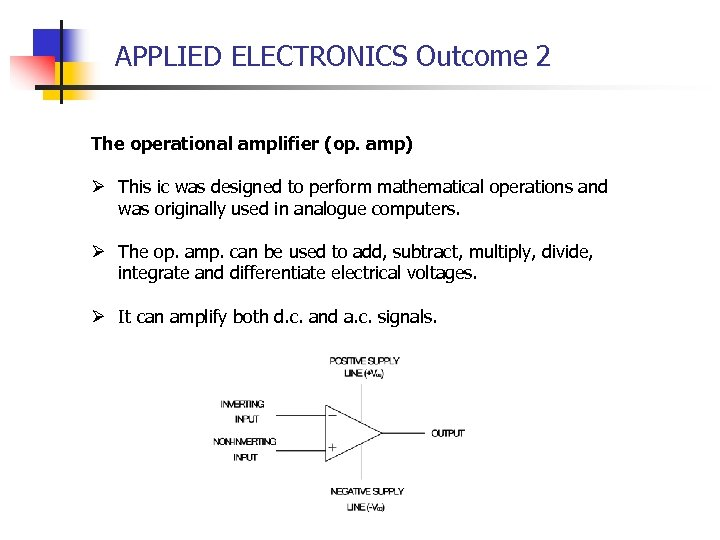APPLIED ELECTRONICS Outcome 2 The operational amplifier (op. amp) Ø This ic was designed to perform mathematical operations and was originally used in analogue computers. Ø The op. amp. can be used to add, subtract, multiply, divide, integrate and differentiate electrical voltages. Ø It can amplify both d. c. and a. c. signals.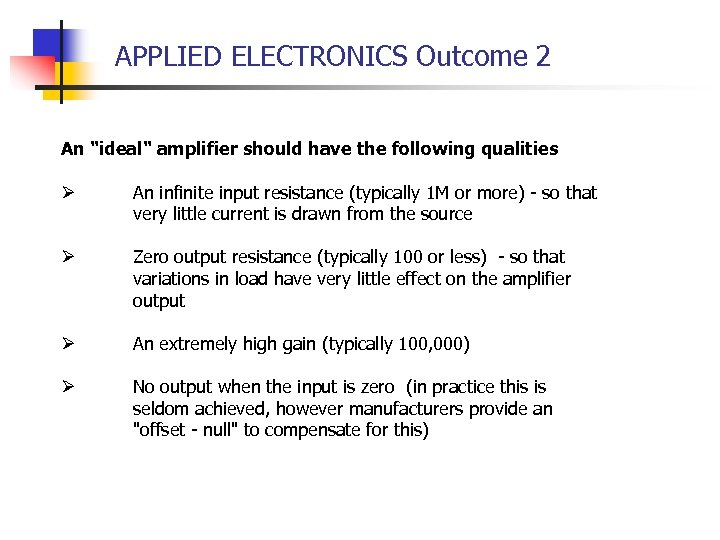APPLIED ELECTRONICS Outcome 2 An "ideal" amplifier should have the following qualities Ø An infinite input resistance (typically 1 M or more) - so that very little current is drawn from the source Ø Zero output resistance (typically 100 or less) - so that variations in load have very little effect on the amplifier output Ø An extremely high gain (typically 100, 000) Ø No output when the input is zero (in practice this is seldom achieved, however manufacturers provide an "offset - null" to compensate for this)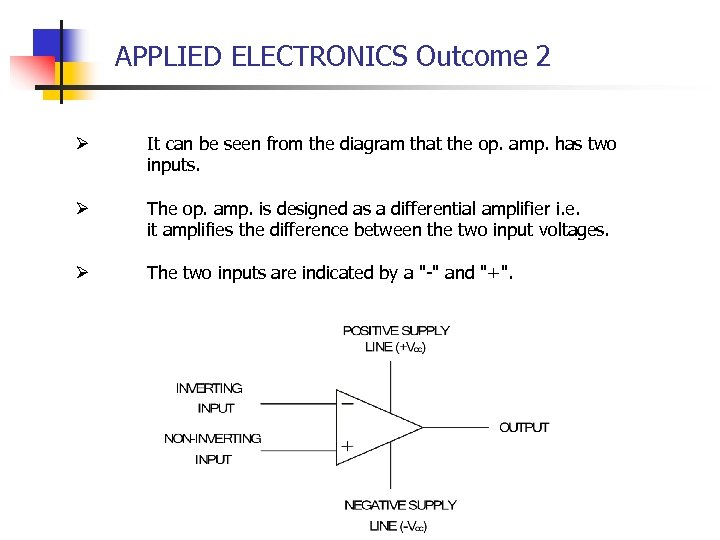APPLIED ELECTRONICS Outcome 2 Ø It can be seen from the diagram that the op. amp. has two inputs. Ø The op. amp. is designed as a differential amplifier i. e. it amplifies the difference between the two input voltages. Ø The two inputs are indicated by a "-" and "+".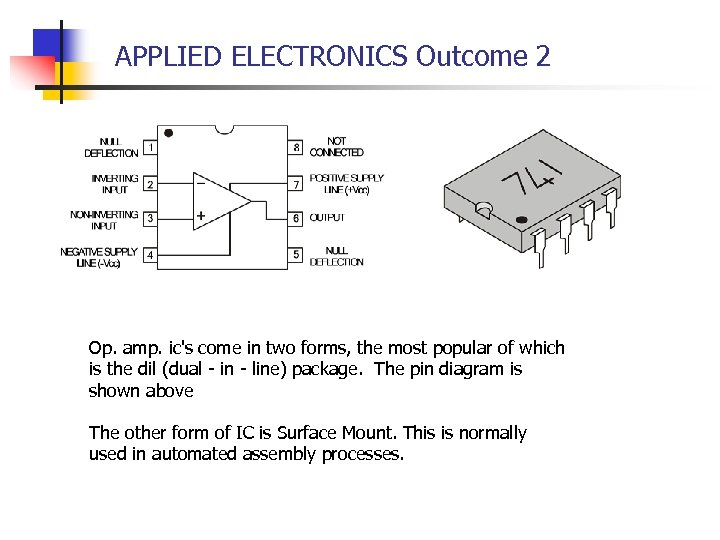APPLIED ELECTRONICS Outcome 2 Op. amp. ic's come in two forms, the most popular of which is the dil (dual - in - line) package. The pin diagram is shown above The other form of IC is Surface Mount. This is normally used in automated assembly processes.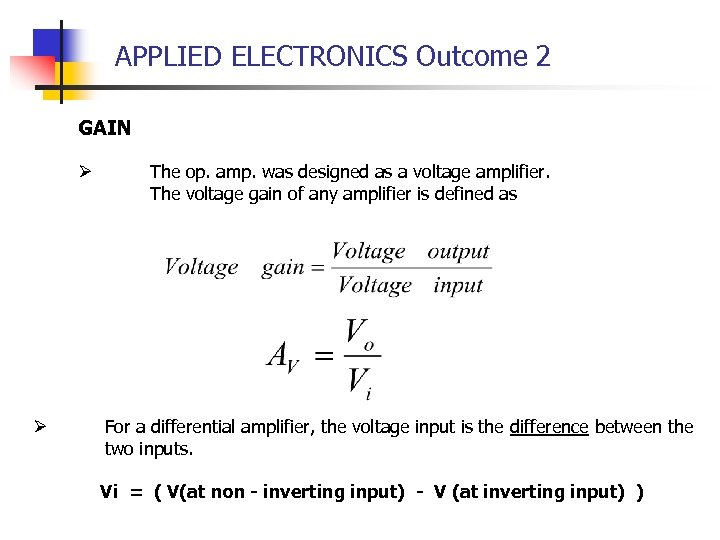APPLIED ELECTRONICS Outcome 2 GAIN Ø Ø The op. amp. was designed as a voltage amplifier. The voltage gain of any amplifier is defined as For a differential amplifier, the voltage input is the difference between the two inputs. Vi = ( V(at non - inverting input) - V (at inverting input) )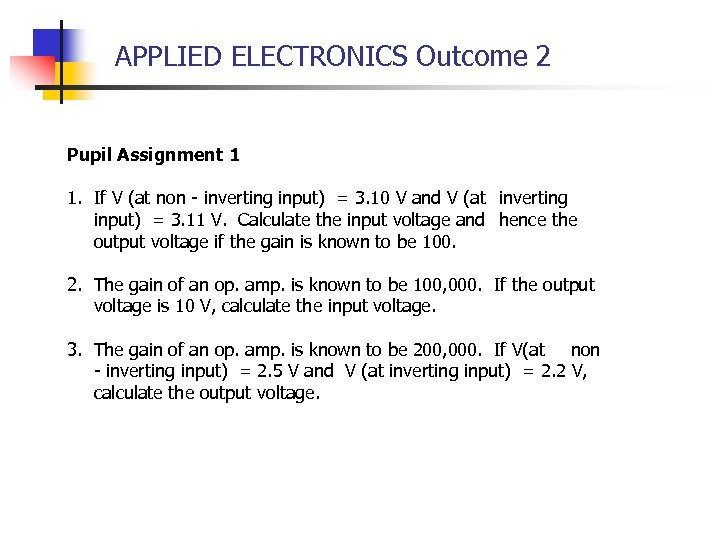APPLIED ELECTRONICS Outcome 2 Pupil Assignment 1 1. If V (at non - inverting input) = 3. 10 V and V (at inverting input) = 3. 11 V. Calculate the input voltage and hence the output voltage if the gain is known to be 100. 2. The gain of an op. amp. is known to be 100, 000. If the output voltage is 10 V, calculate the input voltage. 3. The gain of an op. amp. is known to be 200, 000. If V(at non - inverting input) = 2. 5 V and V (at inverting input) = 2. 2 V, calculate the output voltage.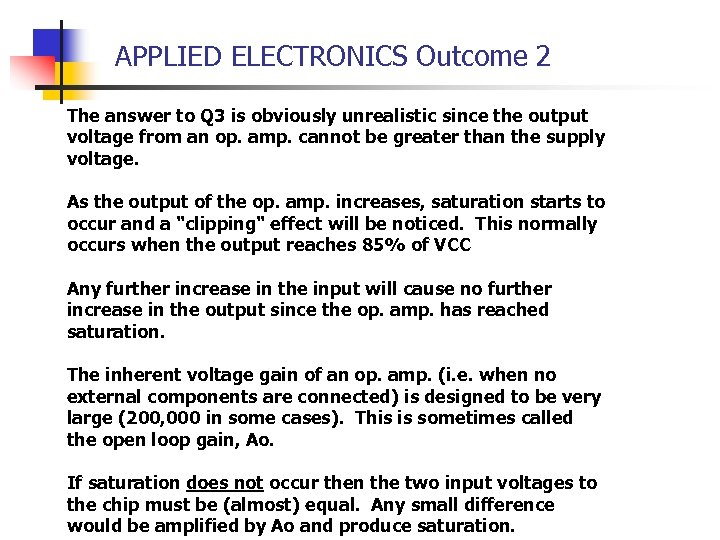APPLIED ELECTRONICS Outcome 2 The answer to Q 3 is obviously unrealistic since the output voltage from an op. amp. cannot be greater than the supply voltage. As the output of the op. amp. increases, saturation starts to occur and a "clipping" effect will be noticed. This normally occurs when the output reaches 85% of VCC Any further increase in the input will cause no further increase in the output since the op. amp. has reached saturation. The inherent voltage gain of an op. amp. (i. e. when no external components are connected) is designed to be very large (200, 000 in some cases). This is sometimes called the open loop gain, Ao. If saturation does not occur then the two input voltages to the chip must be (almost) equal. Any small difference would be amplified by Ao and produce saturation.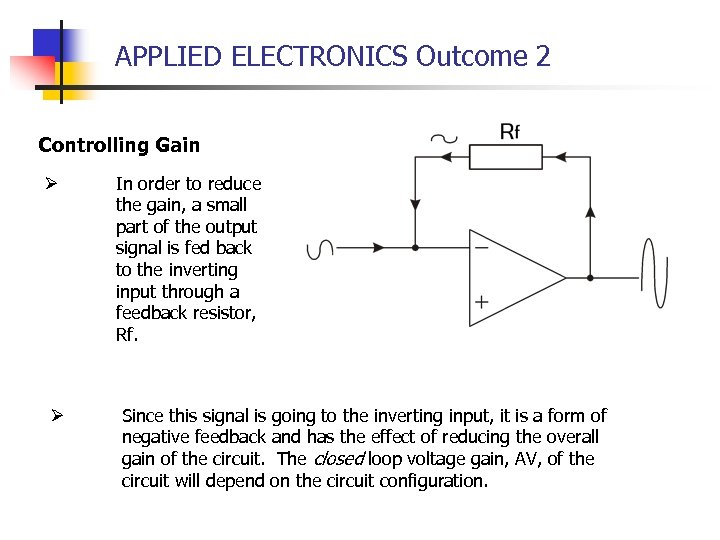APPLIED ELECTRONICS Outcome 2 Controlling Gain Ø Ø In order to reduce the gain, a small part of the output signal is fed back to the inverting input through a feedback resistor, Rf. Since this signal is going to the inverting input, it is a form of negative feedback and has the effect of reducing the overall gain of the circuit. The closed loop voltage gain, AV, of the circuit will depend on the circuit configuration.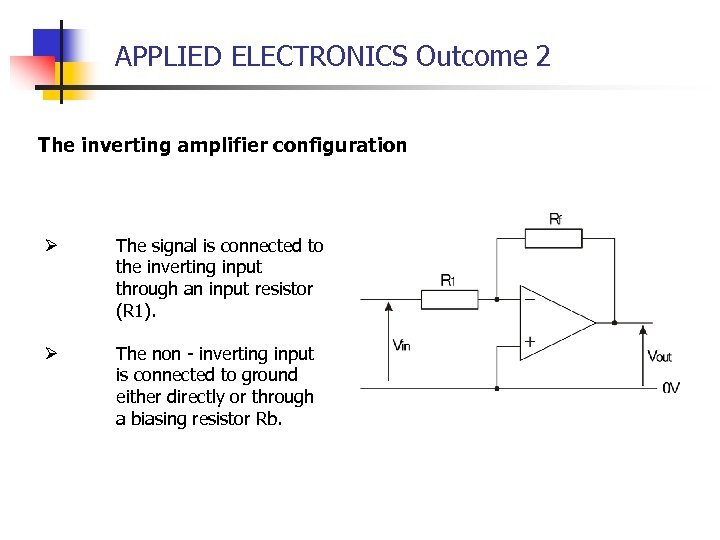APPLIED ELECTRONICS Outcome 2 The inverting amplifier configuration Ø The signal is connected to the inverting input through an input resistor (R 1). Ø The non - inverting input is connected to ground either directly or through a biasing resistor Rb.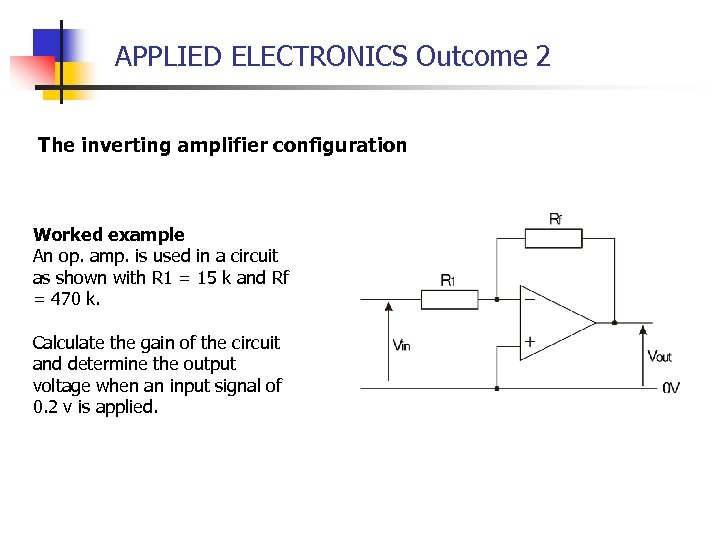APPLIED ELECTRONICS Outcome 2 The inverting amplifier configuration Worked example An op. amp. is used in a circuit as shown with R 1 = 15 k and Rf = 470 k. Calculate the gain of the circuit and determine the output voltage when an input signal of 0. 2 v is applied.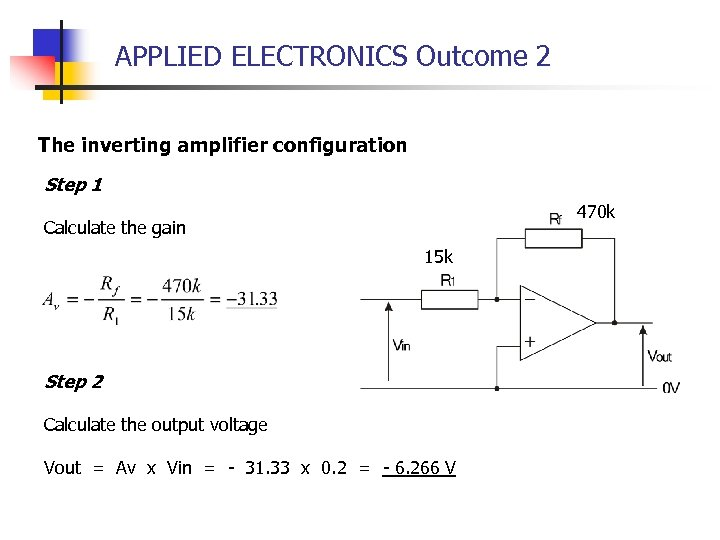APPLIED ELECTRONICS Outcome 2 The inverting amplifier configuration Step 1 470 k Calculate the gain 15 k Step 2 Calculate the output voltage Vout = Av x Vin = - 31. 33 x 0. 2 = - 6. 266 V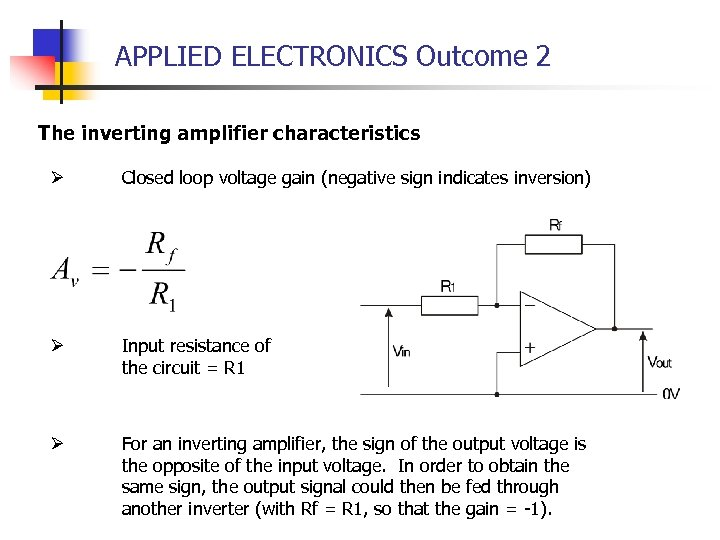APPLIED ELECTRONICS Outcome 2 The inverting amplifier characteristics Ø Closed loop voltage gain (negative sign indicates inversion) Ø Input resistance of the circuit = R 1 Ø For an inverting amplifier, the sign of the output voltage is the opposite of the input voltage. In order to obtain the same sign, the output signal could then be fed through another inverter (with Rf = R 1, so that the gain = -1).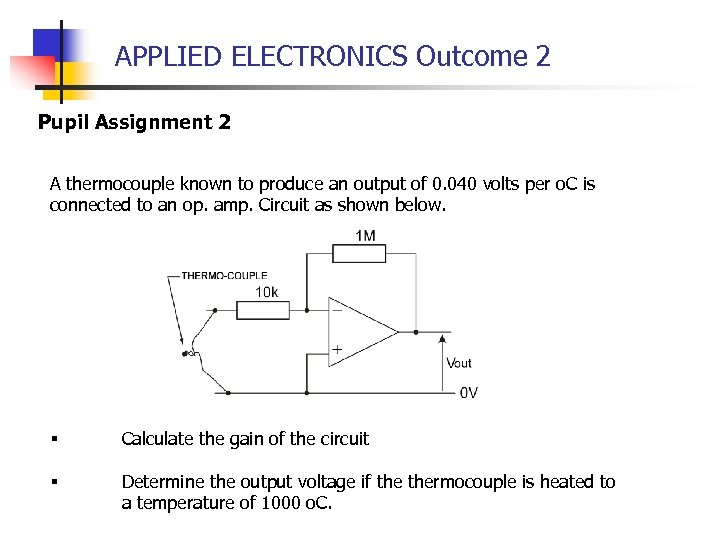APPLIED ELECTRONICS Outcome 2 Pupil Assignment 2 A thermocouple known to produce an output of 0. 040 volts per o. C is connected to an op. amp. Circuit as shown below. § Calculate the gain of the circuit § Determine the output voltage if thermocouple is heated to a temperature of 1000 o. C.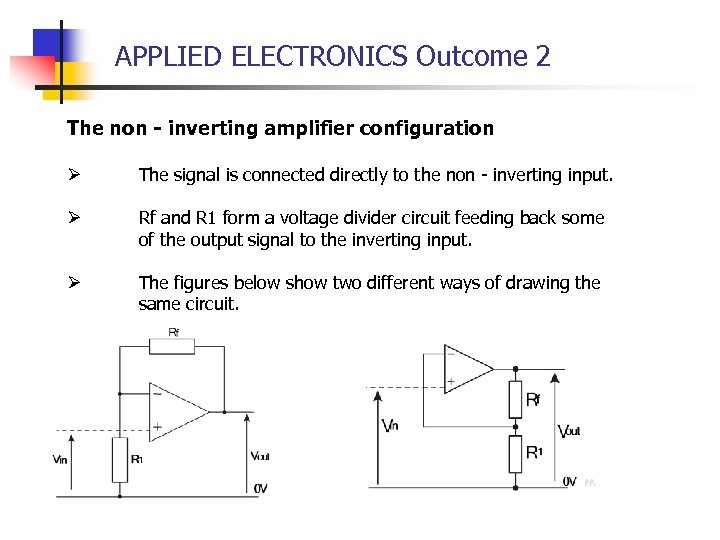APPLIED ELECTRONICS Outcome 2 The non - inverting amplifier configuration Ø The signal is connected directly to the non - inverting input. Ø Rf and R 1 form a voltage divider circuit feeding back some of the output signal to the inverting input. Ø The figures below show two different ways of drawing the same circuit.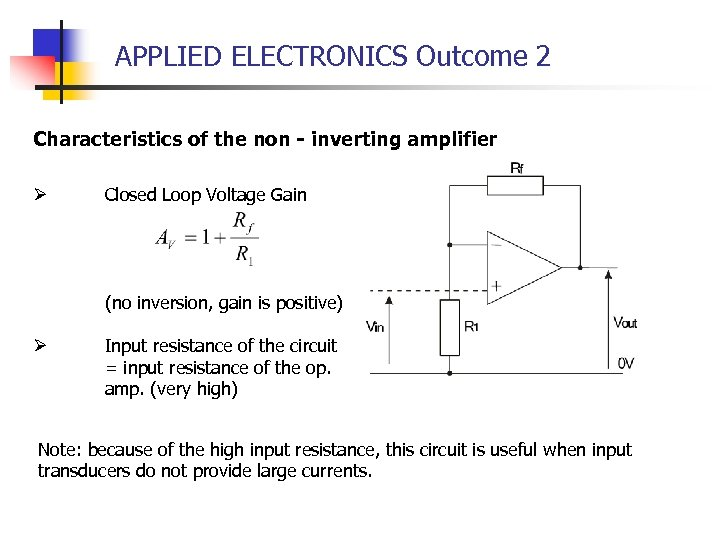APPLIED ELECTRONICS Outcome 2 Characteristics of the non - inverting amplifier Ø Closed Loop Voltage Gain (no inversion, gain is positive) Ø Input resistance of the circuit = input resistance of the op. amp. (very high) Note: because of the high input resistance, this circuit is useful when input transducers do not provide large currents.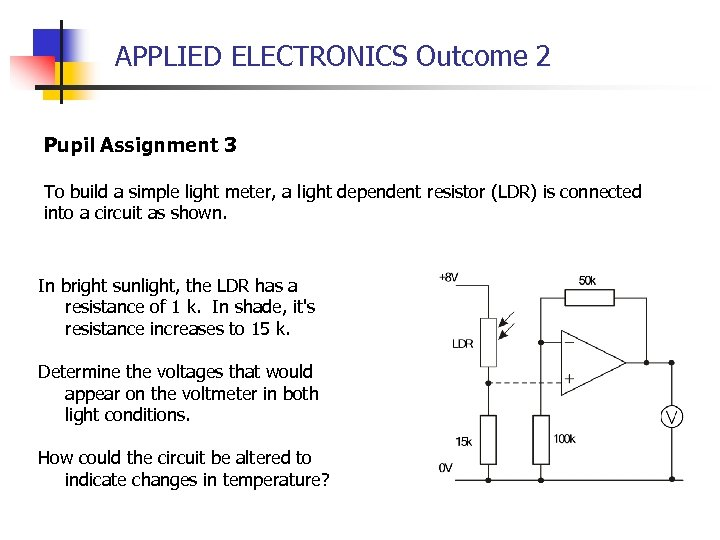APPLIED ELECTRONICS Outcome 2 Pupil Assignment 3 To build a simple light meter, a light dependent resistor (LDR) is connected into a circuit as shown. In bright sunlight, the LDR has a resistance of 1 k. In shade, it's resistance increases to 15 k. Determine the voltages that would appear on the voltmeter in both light conditions. How could the circuit be altered to indicate changes in temperature?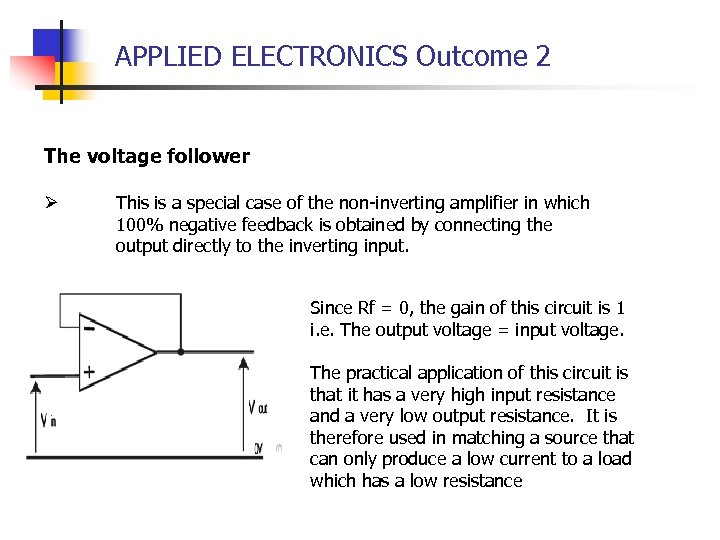APPLIED ELECTRONICS Outcome 2 The voltage follower Ø This is a special case of the non-inverting amplifier in which 100% negative feedback is obtained by connecting the output directly to the inverting input. Since Rf = 0, the gain of this circuit is 1 i. e. The output voltage = input voltage. The practical application of this circuit is that it has a very high input resistance and a very low output resistance. It is therefore used in matching a source that can only produce a low current to a load which has a low resistance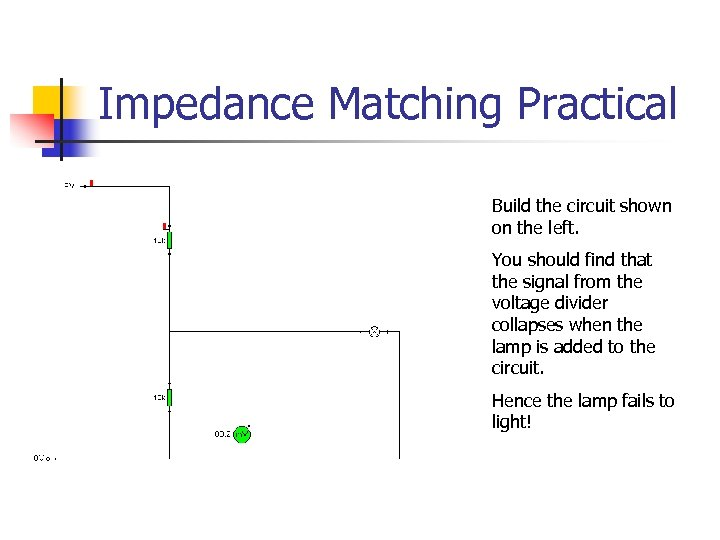Impedance Matching Practical Build the circuit shown on the left. You should find that the signal from the voltage divider collapses when the lamp is added to the circuit. Hence the lamp fails to light!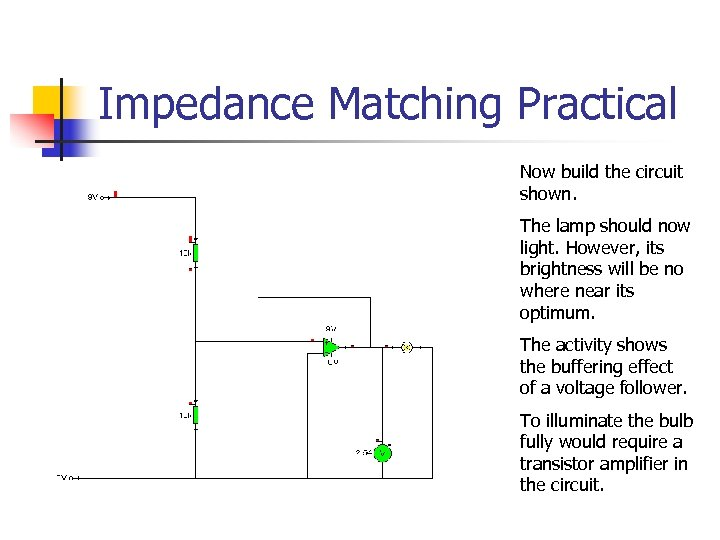Impedance Matching Practical Now build the circuit shown. The lamp should now light. However, its brightness will be no where near its optimum. The activity shows the buffering effect of a voltage follower. To illuminate the bulb fully would require a transistor amplifier in the circuit.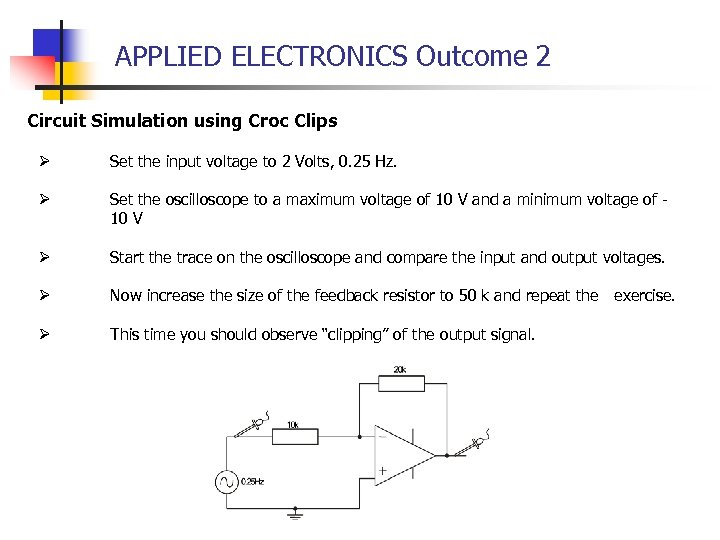APPLIED ELECTRONICS Outcome 2 Circuit Simulation using Croc Clips Ø Set the input voltage to 2 Volts, 0. 25 Hz. Ø Set the oscilloscope to a maximum voltage of 10 V and a minimum voltage of 10 V Ø Start the trace on the oscilloscope and compare the input and output voltages. Ø Now increase the size of the feedback resistor to 50 k and repeat the exercise. Ø This time you should observe “clipping” of the output signal.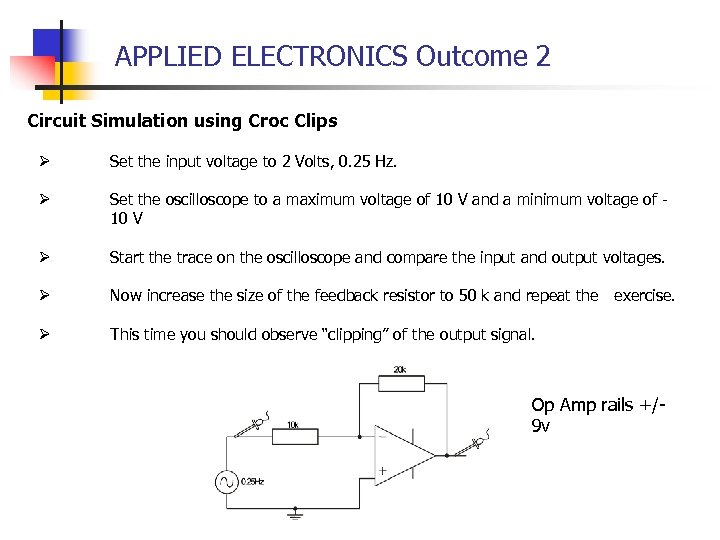APPLIED ELECTRONICS Outcome 2 Circuit Simulation using Croc Clips Ø Set the input voltage to 2 Volts, 0. 25 Hz. Ø Set the oscilloscope to a maximum voltage of 10 V and a minimum voltage of 10 V Ø Start the trace on the oscilloscope and compare the input and output voltages. Ø Now increase the size of the feedback resistor to 50 k and repeat the exercise. Ø This time you should observe “clipping” of the output signal. Op Amp rails +/9 v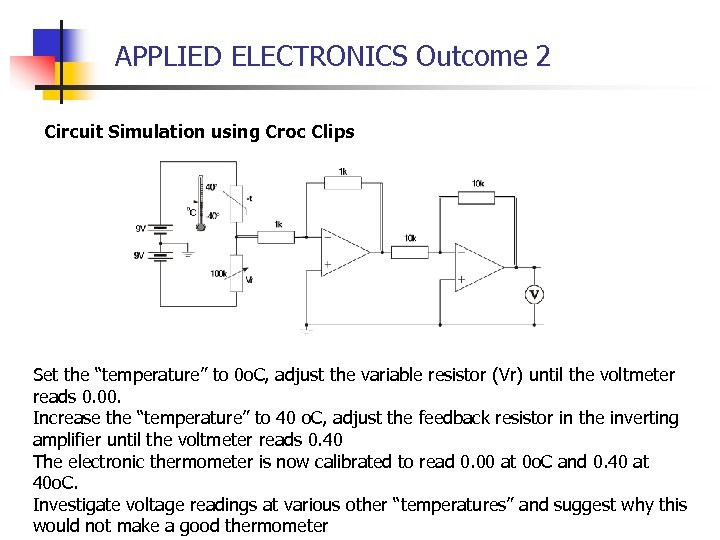APPLIED ELECTRONICS Outcome 2 Circuit Simulation using Croc Clips Set the “temperature” to 0 o. C, adjust the variable resistor (Vr) until the voltmeter reads 0. 00. Increase the “temperature” to 40 o. C, adjust the feedback resistor in the inverting amplifier until the voltmeter reads 0. 40 The electronic thermometer is now calibrated to read 0. 00 at 0 o. C and 0. 40 at 40 o. C. Investigate voltage readings at various other “temperatures” and suggest why this would not make a good thermometer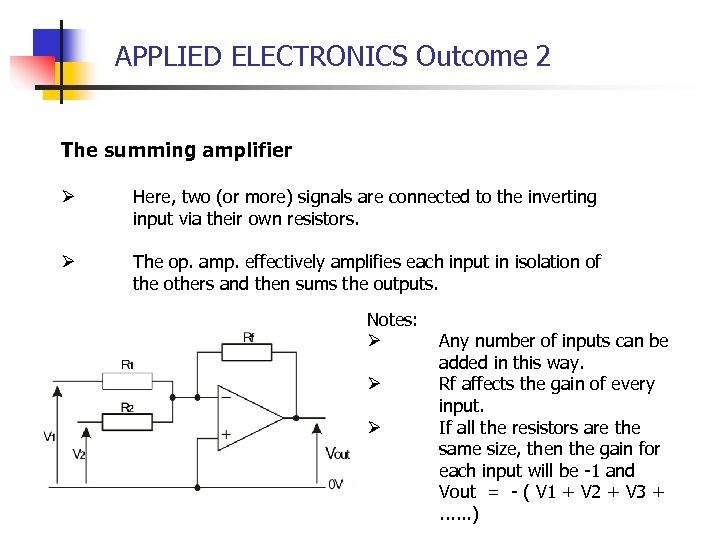APPLIED ELECTRONICS Outcome 2 The summing amplifier Ø Here, two (or more) signals are connected to the inverting input via their own resistors. Ø The op. amp. effectively amplifies each input in isolation of the others and then sums the outputs. Notes: Ø Ø Ø Any number of inputs can be added in this way. Rf affects the gain of every input. If all the resistors are the same size, then the gain for each input will be -1 and Vout = - ( V 1 + V 2 + V 3 +. . . )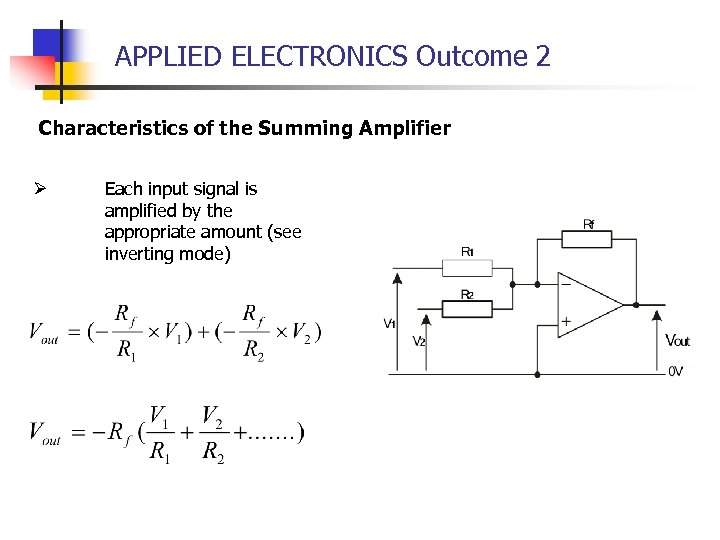APPLIED ELECTRONICS Outcome 2 Characteristics of the Summing Amplifier Ø Each input signal is amplified by the appropriate amount (see inverting mode)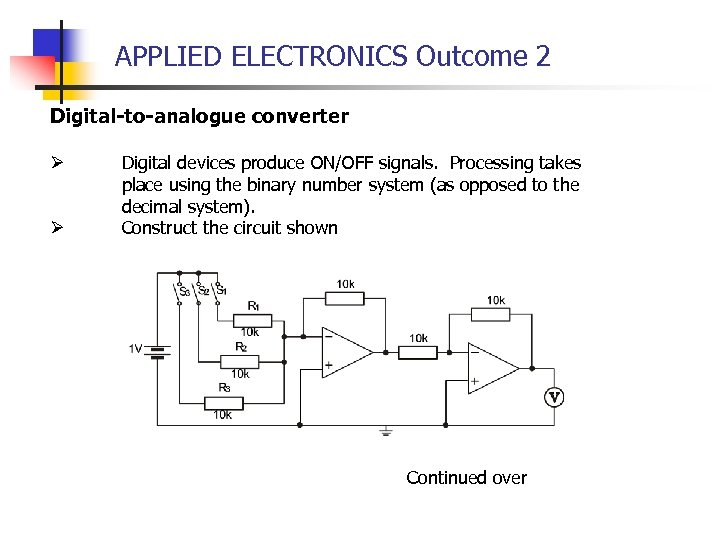APPLIED ELECTRONICS Outcome 2 Digital-to-analogue converter Ø Ø Digital devices produce ON/OFF signals. Processing takes place using the binary number system (as opposed to the decimal system). Construct the circuit shown Continued over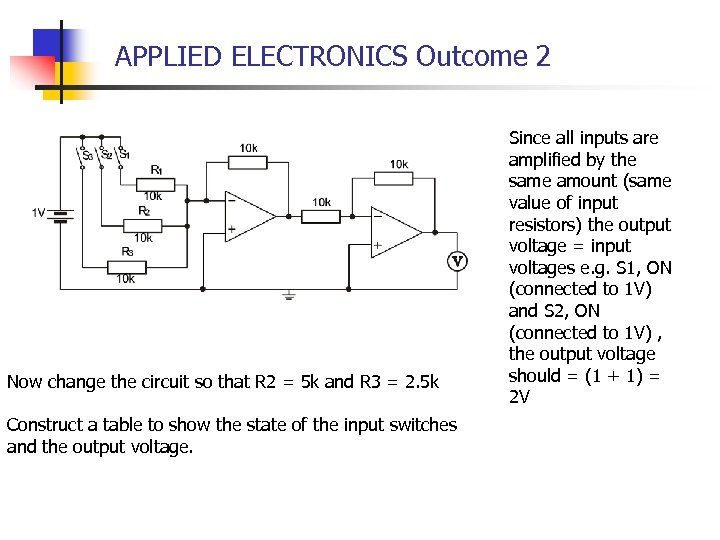APPLIED ELECTRONICS Outcome 2 Now change the circuit so that R 2 = 5 k and R 3 = 2. 5 k Construct a table to show the state of the input switches and the output voltage. Since all inputs are amplified by the same amount (same value of input resistors) the output voltage = input voltages e. g. S 1, ON (connected to 1 V) and S 2, ON (connected to 1 V) , the output voltage should = (1 + 1) = 2 V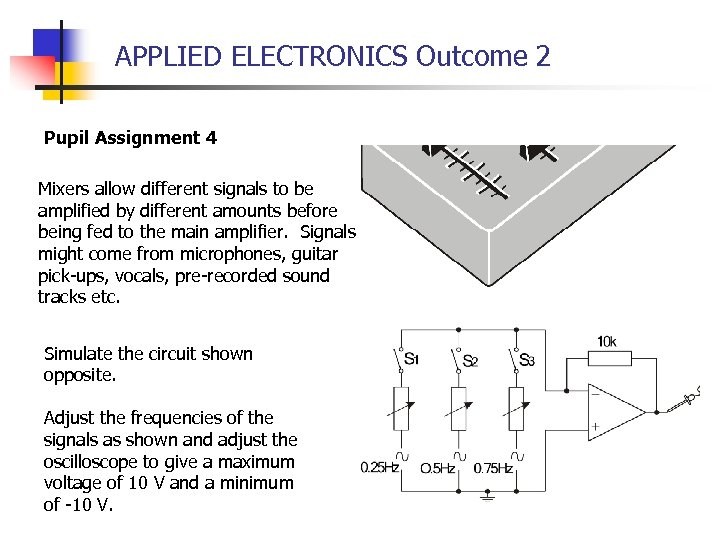APPLIED ELECTRONICS Outcome 2 Pupil Assignment 4 Mixers allow different signals to be amplified by different amounts before being fed to the main amplifier. Signals might come from microphones, guitar pick-ups, vocals, pre-recorded sound tracks etc. Simulate the circuit shown opposite. Adjust the frequencies of the signals as shown and adjust the oscilloscope to give a maximum voltage of 10 V and a minimum of -10 V.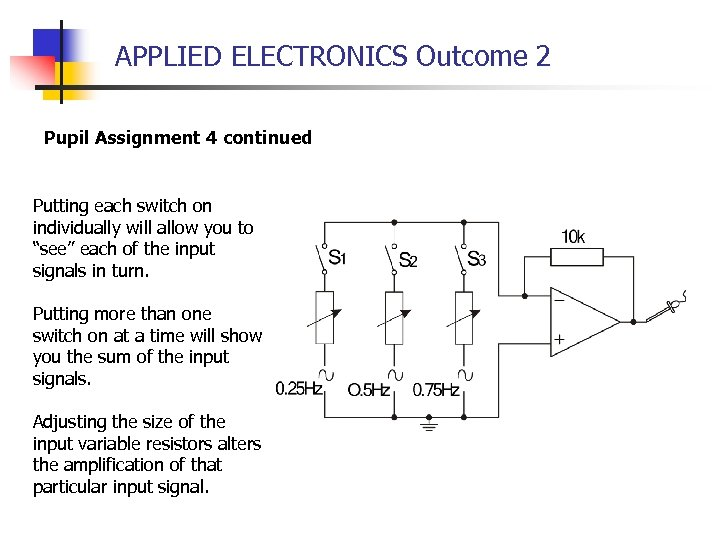APPLIED ELECTRONICS Outcome 2 Pupil Assignment 4 continued Putting each switch on individually will allow you to “see” each of the input signals in turn. Putting more than one switch on at a time will show you the sum of the input signals. Adjusting the size of the input variable resistors alters the amplification of that particular input signal.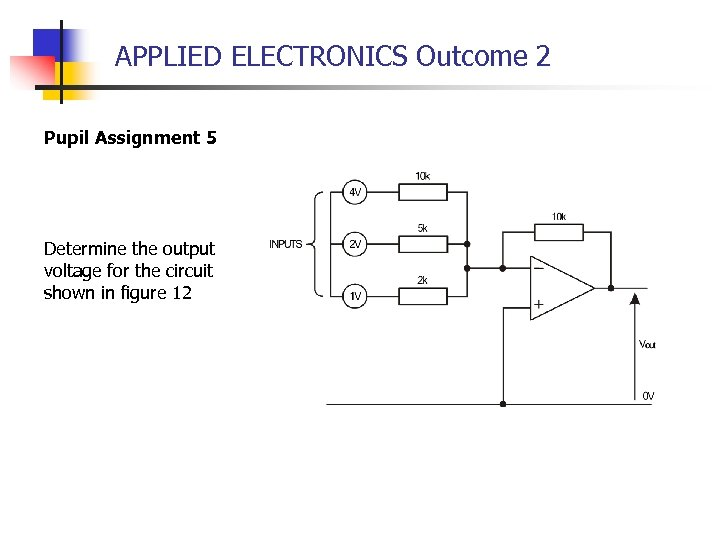APPLIED ELECTRONICS Outcome 2 Pupil Assignment 5 Determine the output voltage for the circuit shown in figure 12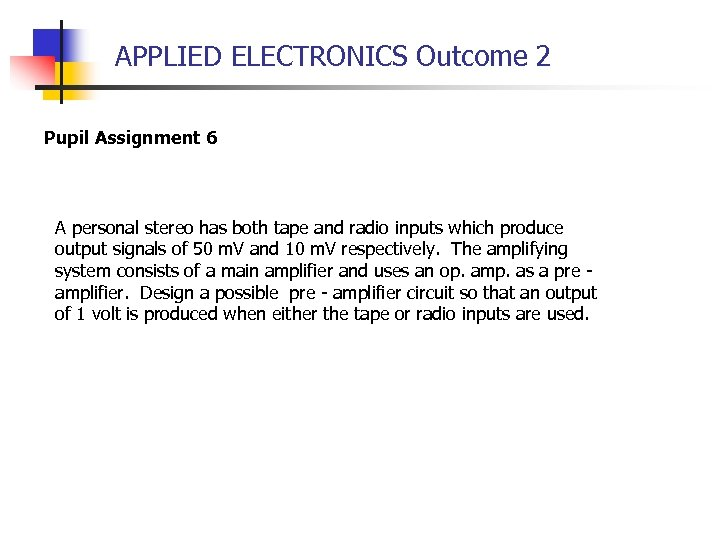APPLIED ELECTRONICS Outcome 2 Pupil Assignment 6 A personal stereo has both tape and radio inputs which produce output signals of 50 m. V and 10 m. V respectively. The amplifying system consists of a main amplifier and uses an op. amp. as a pre amplifier. Design a possible pre - amplifier circuit so that an output of 1 volt is produced when either the tape or radio inputs are used.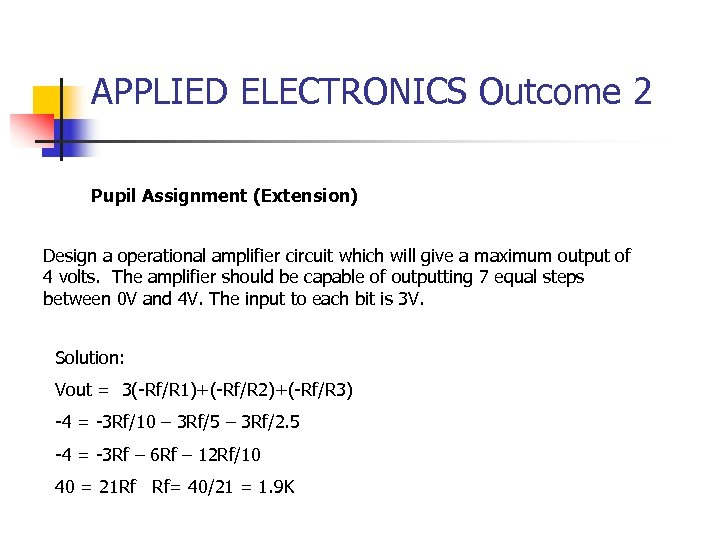APPLIED ELECTRONICS Outcome 2 Pupil Assignment (Extension) Design a operational amplifier circuit which will give a maximum output of 4 volts. The amplifier should be capable of outputting 7 equal steps between 0 V and 4 V. The input to each bit is 3 V. Solution: Vout = 3(-Rf/R 1)+(-Rf/R 2)+(-Rf/R 3) -4 = -3 Rf/10 – 3 Rf/5 – 3 Rf/2. 5 -4 = -3 Rf – 6 Rf – 12 Rf/10 40 = 21 Rf Rf= 40/21 = 1. 9 K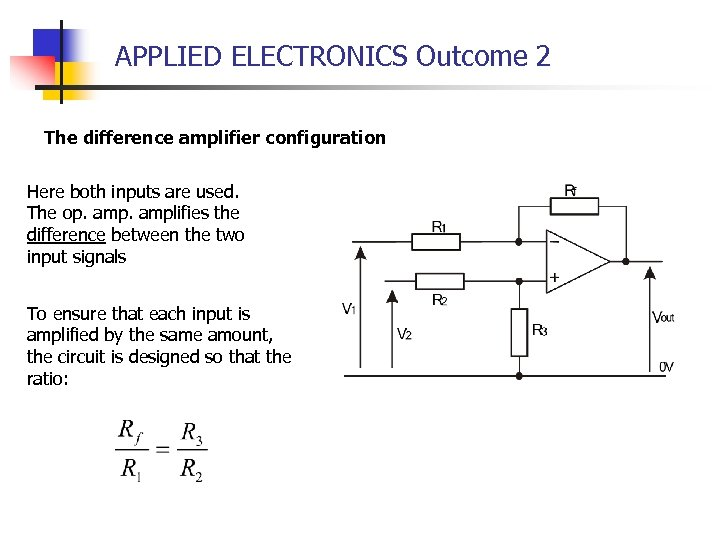APPLIED ELECTRONICS Outcome 2 The difference amplifier configuration Here both inputs are used. The op. amplifies the difference between the two input signals To ensure that each input is amplified by the same amount, the circuit is designed so that the ratio: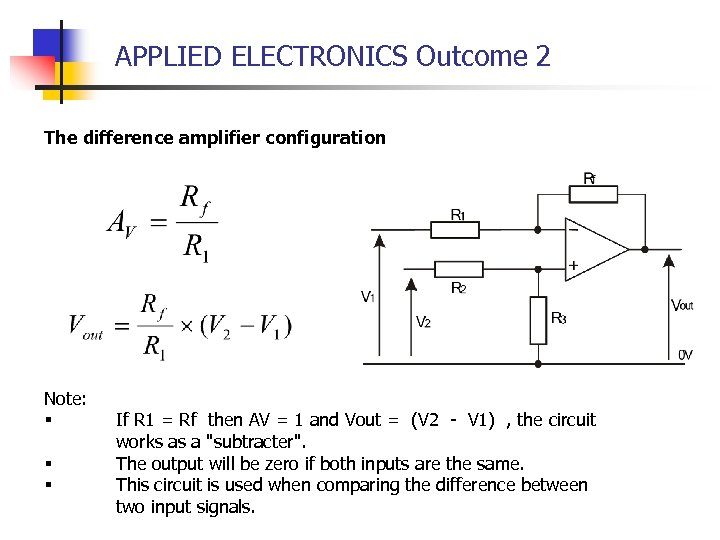APPLIED ELECTRONICS Outcome 2 The difference amplifier configuration Note: § § § If R 1 = Rf then AV = 1 and Vout = (V 2 - V 1) , the circuit works as a "subtracter". The output will be zero if both inputs are the same. This circuit is used when comparing the difference between two input signals.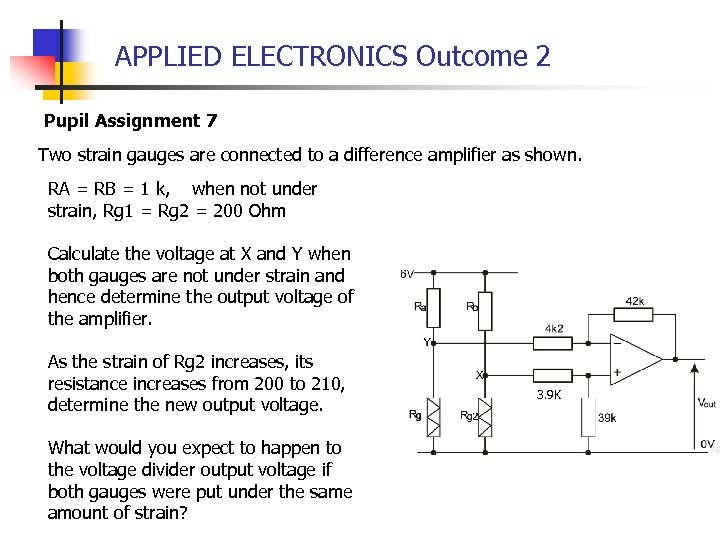APPLIED ELECTRONICS Outcome 2 Pupil Assignment 7 Two strain gauges are connected to a difference amplifier as shown. RA = RB = 1 k, when not under strain, Rg 1 = Rg 2 = 200 Ohm Calculate the voltage at X and Y when both gauges are not under strain and hence determine the output voltage of the amplifier. As the strain of Rg 2 increases, its resistance increases from 200 to 210, determine the new output voltage. What would you expect to happen to the voltage divider output voltage if both gauges were put under the same amount of strain? 3. 9 K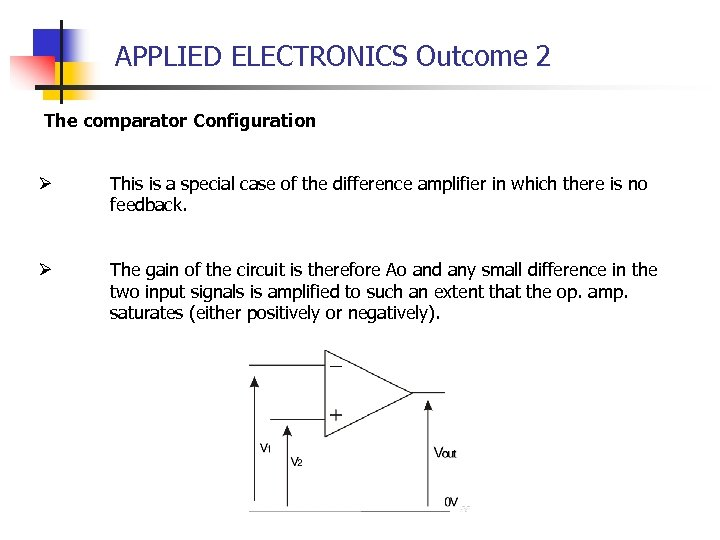APPLIED ELECTRONICS Outcome 2 The comparator Configuration Ø This is a special case of the difference amplifier in which there is no feedback. Ø The gain of the circuit is therefore Ao and any small difference in the two input signals is amplified to such an extent that the op. amp. saturates (either positively or negatively).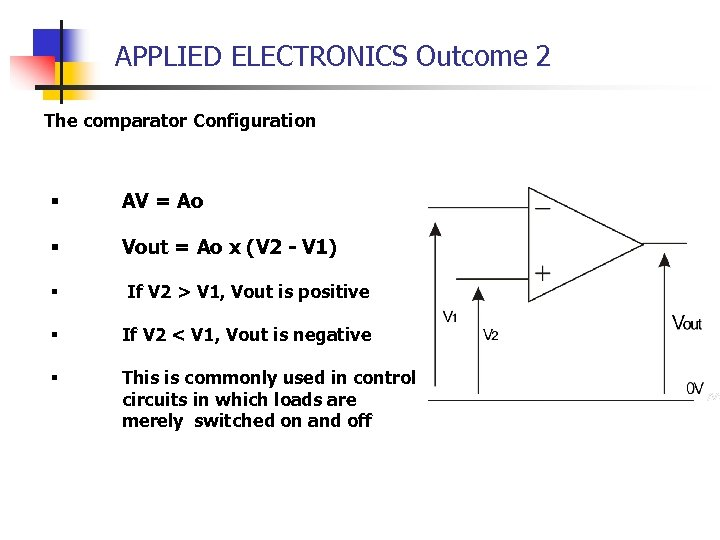APPLIED ELECTRONICS Outcome 2 The comparator Configuration § AV = Ao § Vout = Ao x (V 2 - V 1) § If V 2 > V 1, Vout is positive § If V 2 < V 1, Vout is negative § This is commonly used in control circuits in which loads are merely switched on and off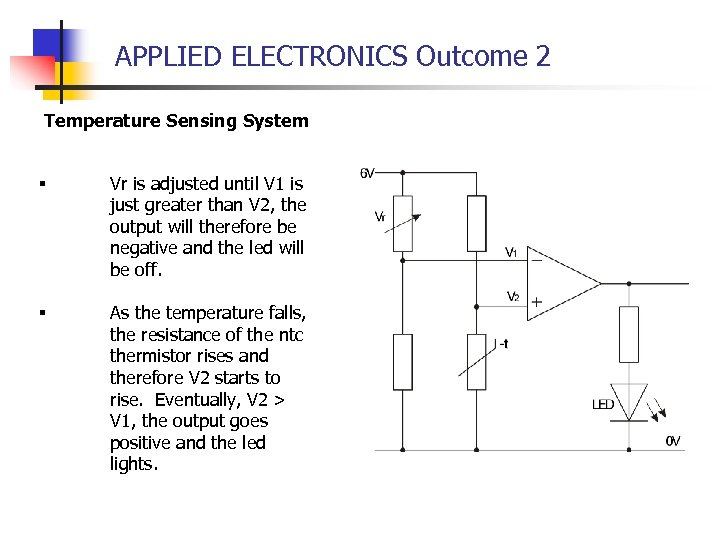APPLIED ELECTRONICS Outcome 2 Temperature Sensing System § Vr is adjusted until V 1 is just greater than V 2, the output will therefore be negative and the led will be off. § As the temperature falls, the resistance of the ntc thermistor rises and therefore V 2 starts to rise. Eventually, V 2 > V 1, the output goes positive and the led lights.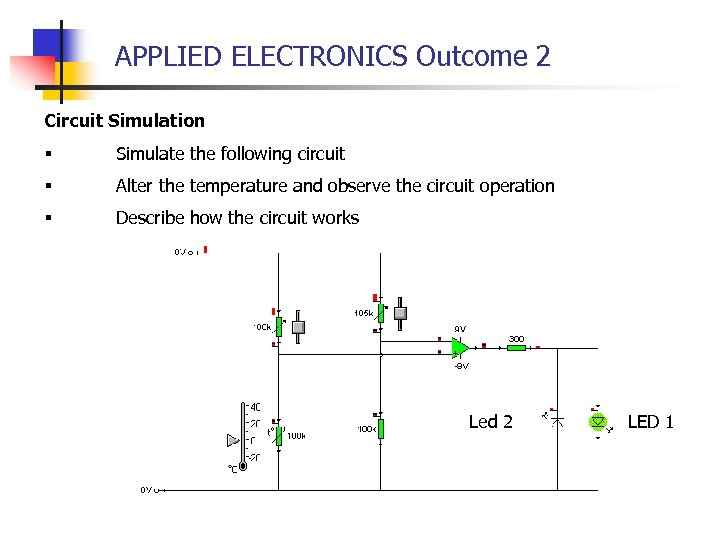APPLIED ELECTRONICS Outcome 2 Circuit Simulation § Simulate the following circuit § Alter the temperature and observe the circuit operation § Describe how the circuit works Led 2 LED 1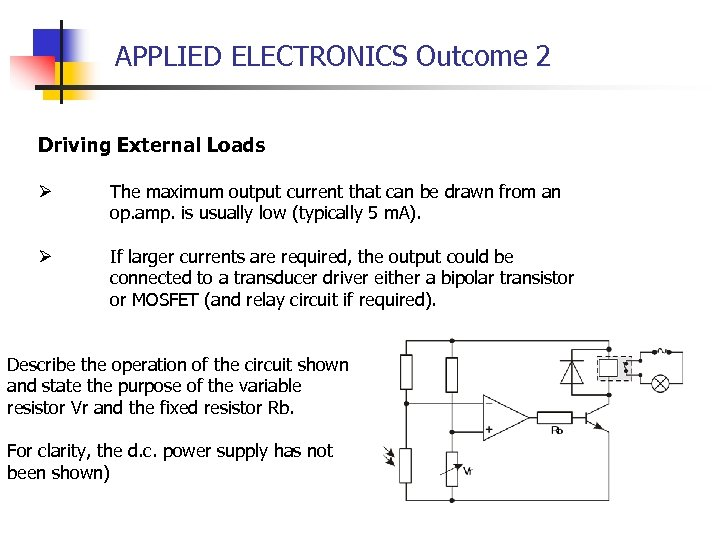APPLIED ELECTRONICS Outcome 2 Driving External Loads Ø The maximum output current that can be drawn from an op. amp. is usually low (typically 5 m. A). Ø If larger currents are required, the output could be connected to a transducer driver either a bipolar transistor or MOSFET (and relay circuit if required). Describe the operation of the circuit shown and state the purpose of the variable resistor Vr and the fixed resistor Rb. For clarity, the d. c. power supply has not been shown)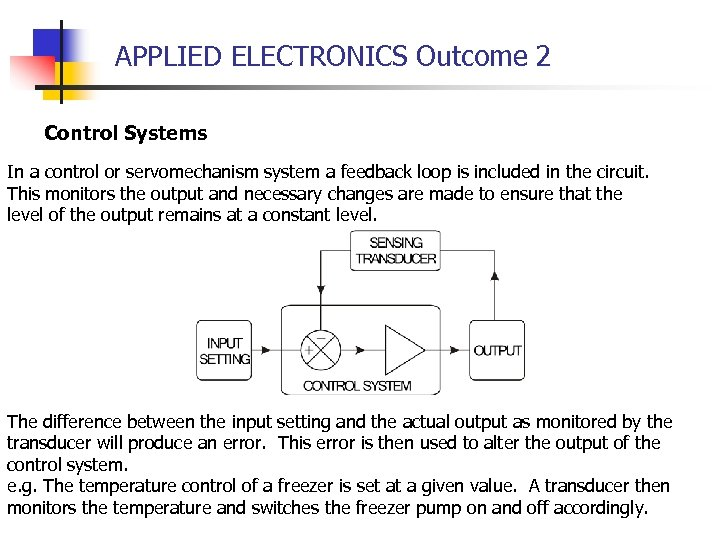APPLIED ELECTRONICS Outcome 2 Control Systems In a control or servomechanism system a feedback loop is included in the circuit. This monitors the output and necessary changes are made to ensure that the level of the output remains at a constant level. The difference between the input setting and the actual output as monitored by the transducer will produce an error. This error is then used to alter the output of the control system. e. g. The temperature control of a freezer is set at a given value. A transducer then monitors the temperature and switches the freezer pump on and off accordingly.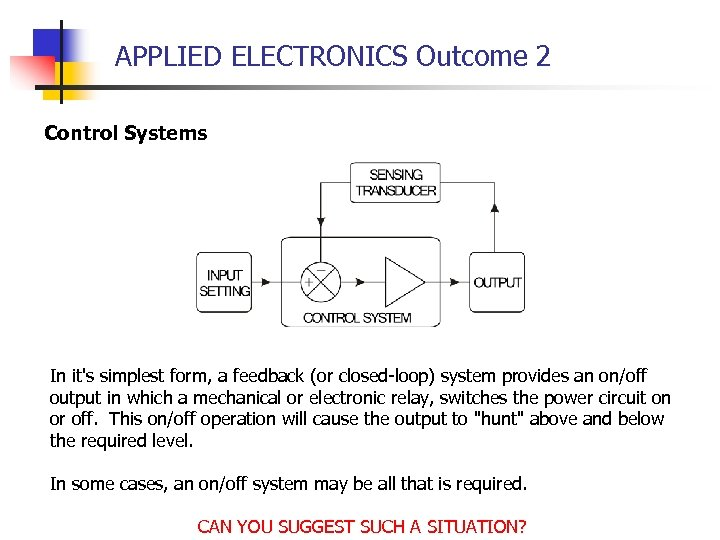APPLIED ELECTRONICS Outcome 2 Control Systems In it's simplest form, a feedback (or closed-loop) system provides an on/off output in which a mechanical or electronic relay, switches the power circuit on or off. This on/off operation will cause the output to "hunt" above and below the required level. In some cases, an on/off system may be all that is required. CAN YOU SUGGEST SUCH A SITUATION?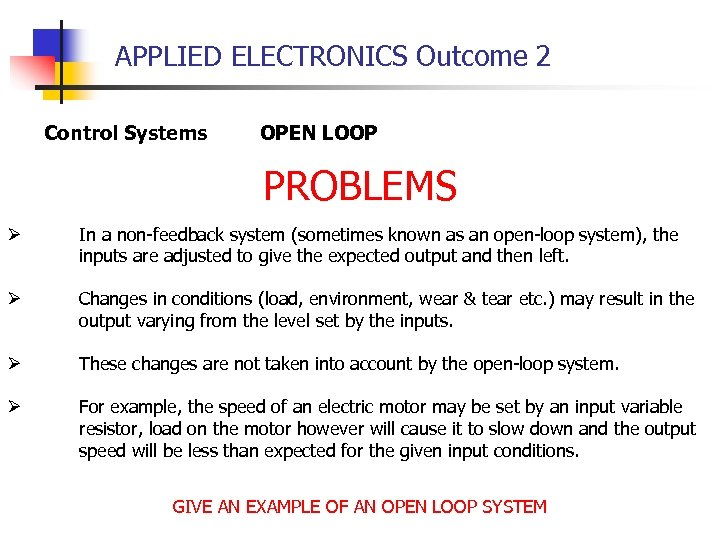APPLIED ELECTRONICS Outcome 2 Control Systems OPEN LOOP PROBLEMS Ø In a non-feedback system (sometimes known as an open-loop system), the inputs are adjusted to give the expected output and then left. Ø Changes in conditions (load, environment, wear & tear etc. ) may result in the output varying from the level set by the inputs. Ø These changes are not taken into account by the open-loop system. Ø For example, the speed of an electric motor may be set by an input variable resistor, load on the motor however will cause it to slow down and the output speed will be less than expected for the given input conditions. GIVE AN EXAMPLE OF AN OPEN LOOP SYSTEM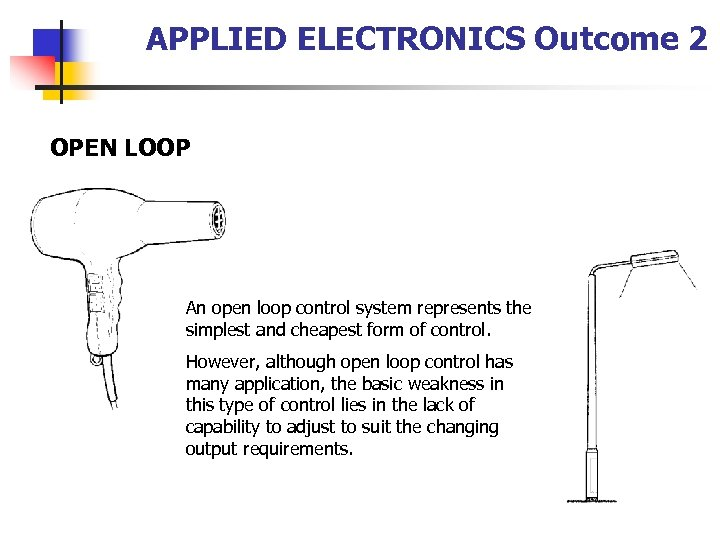APPLIED ELECTRONICS Outcome 2 OPEN LOOP An open loop control system represents the simplest and cheapest form of control. However, although open loop control has many application, the basic weakness in this type of control lies in the lack of capability to adjust to suit the changing output requirements.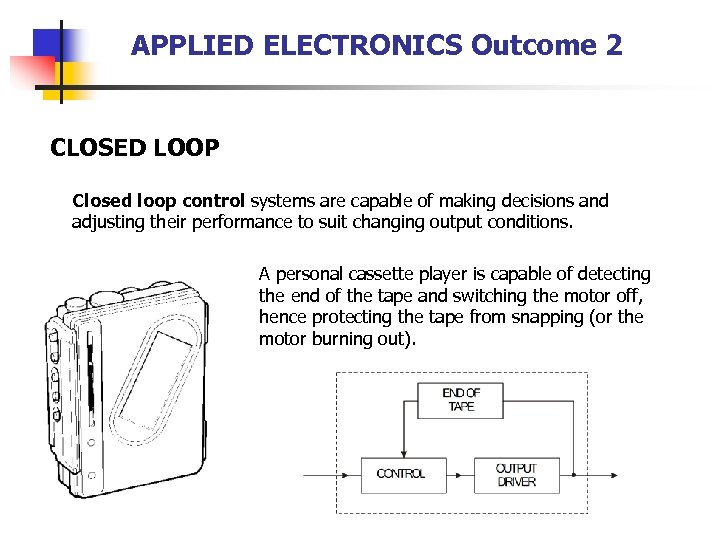APPLIED ELECTRONICS Outcome 2 CLOSED LOOP Closed loop control systems are capable of making decisions and adjusting their performance to suit changing output conditions. A personal cassette player is capable of detecting the end of the tape and switching the motor off, hence protecting the tape from snapping (or the motor burning out).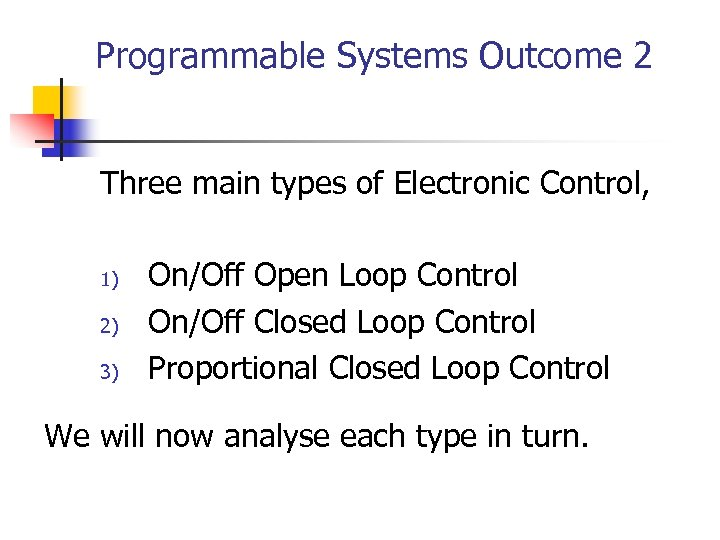Programmable Systems Outcome 2 Three main types of Electronic Control, 1) 2) 3) On/Off Open Loop Control On/Off Closed Loop Control Proportional Closed Loop Control We will now analyse each type in turn.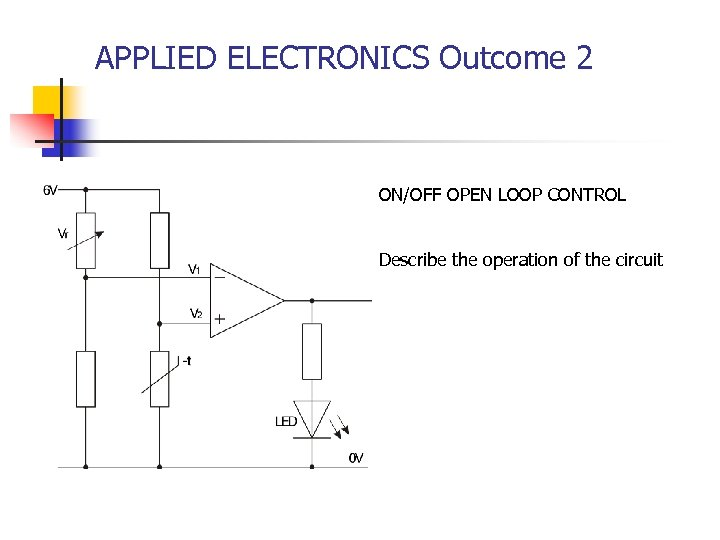APPLIED ELECTRONICS Outcome 2 ON/OFF OPEN LOOP CONTROL Describe the operation of the circuit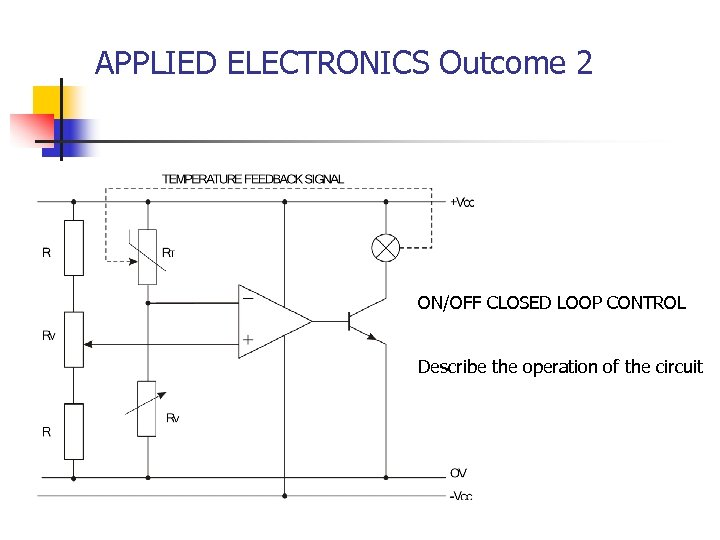APPLIED ELECTRONICS Outcome 2 ON/OFF CLOSED LOOP CONTROL Describe the operation of the circuit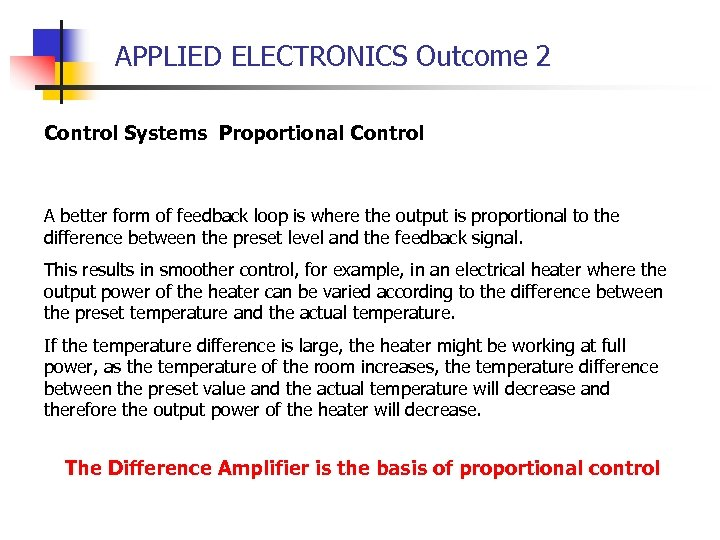APPLIED ELECTRONICS Outcome 2 Control Systems Proportional Control A better form of feedback loop is where the output is proportional to the difference between the preset level and the feedback signal. This results in smoother control, for example, in an electrical heater where the output power of the heater can be varied according to the difference between the preset temperature and the actual temperature. If the temperature difference is large, the heater might be working at full power, as the temperature of the room increases, the temperature difference between the preset value and the actual temperature will decrease and therefore the output power of the heater will decrease. The Difference Amplifier is the basis of proportional control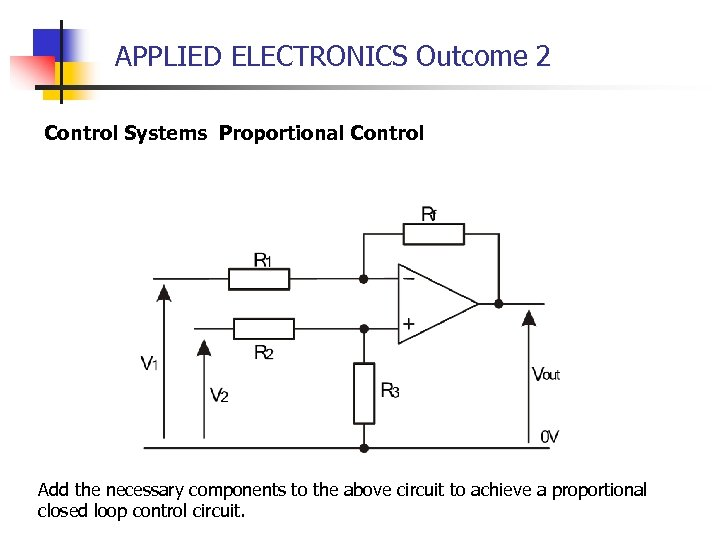APPLIED ELECTRONICS Outcome 2 Control Systems Proportional Control Add the necessary components to the above circuit to achieve a proportional closed loop control circuit.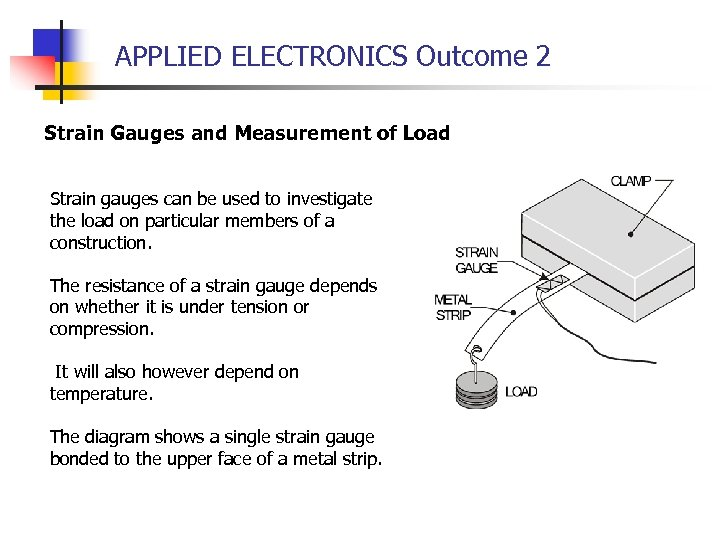APPLIED ELECTRONICS Outcome 2 Strain Gauges and Measurement of Load Strain gauges can be used to investigate the load on particular members of a construction. The resistance of a strain gauge depends on whether it is under tension or compression. It will also however depend on temperature. The diagram shows a single strain gauge bonded to the upper face of a metal strip.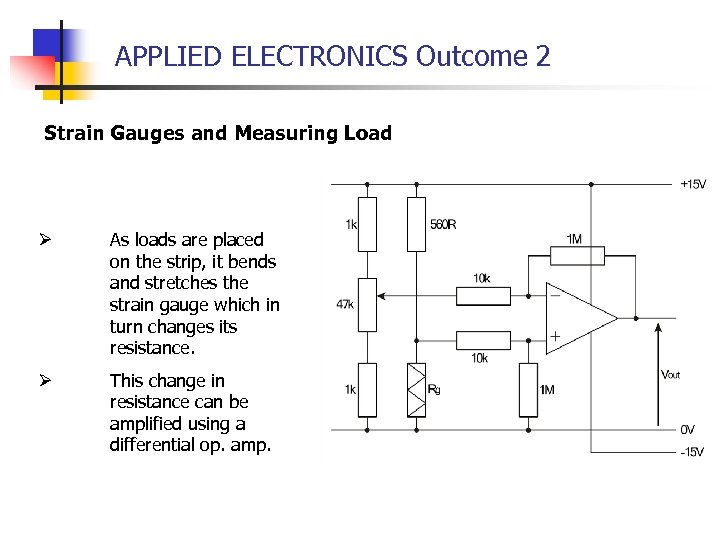APPLIED ELECTRONICS Outcome 2 Strain Gauges and Measuring Load Ø As loads are placed on the strip, it bends and stretches the strain gauge which in turn changes its resistance. Ø This change in resistance can be amplified using a differential op. amp.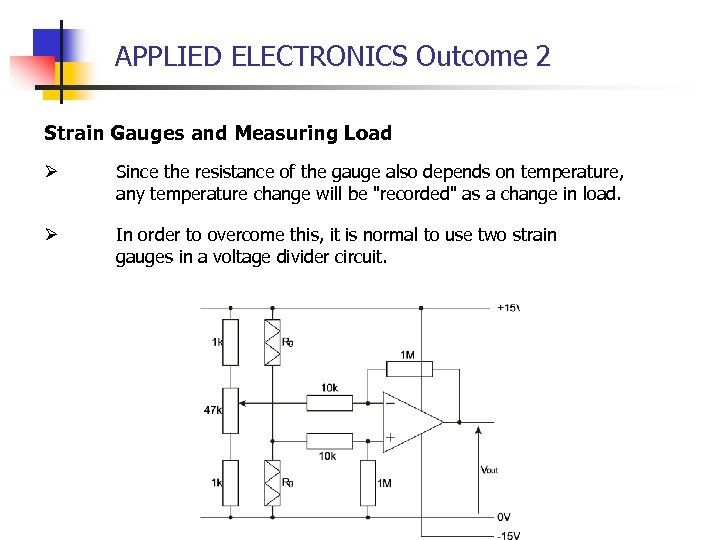APPLIED ELECTRONICS Outcome 2 Strain Gauges and Measuring Load Ø Since the resistance of the gauge also depends on temperature, any temperature change will be "recorded" as a change in load. Ø In order to overcome this, it is normal to use two strain gauges in a voltage divider circuit.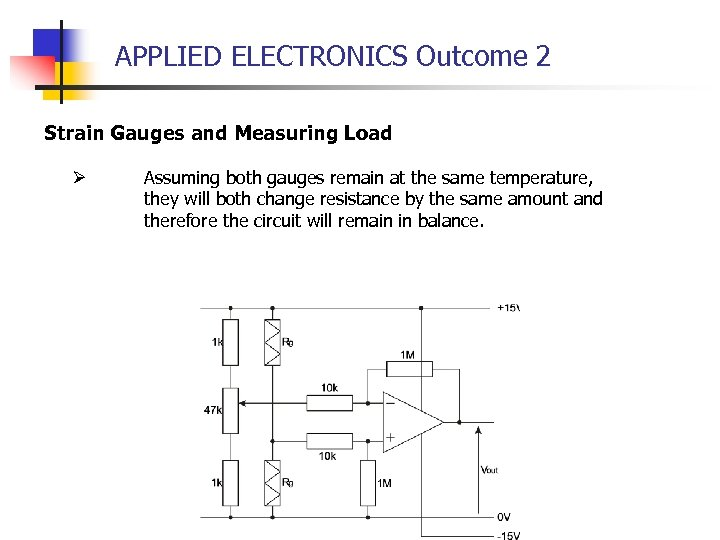APPLIED ELECTRONICS Outcome 2 Strain Gauges and Measuring Load Ø Assuming both gauges remain at the same temperature, they will both change resistance by the same amount and therefore the circuit will remain in balance.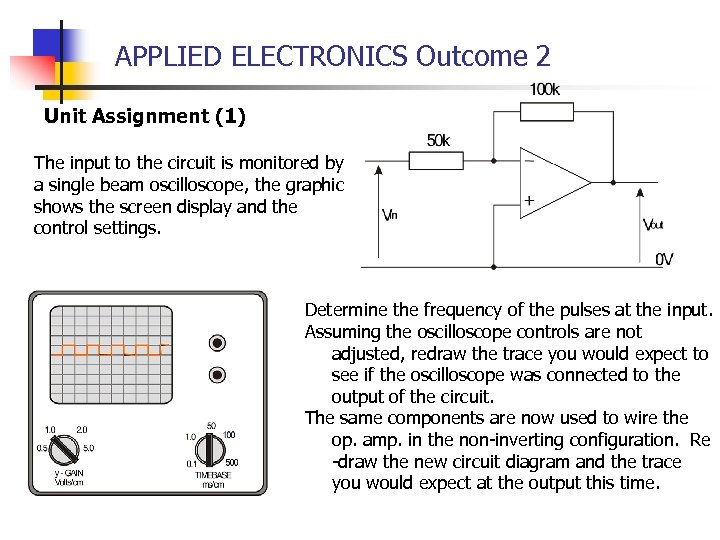APPLIED ELECTRONICS Outcome 2 Unit Assignment (1) The input to the circuit is monitored by a single beam oscilloscope, the graphic shows the screen display and the control settings. Determine the frequency of the pulses at the input. Assuming the oscilloscope controls are not adjusted, redraw the trace you would expect to see if the oscilloscope was connected to the output of the circuit. The same components are now used to wire the op. amp. in the non-inverting configuration. Re -draw the new circuit diagram and the trace you would expect at the output this time.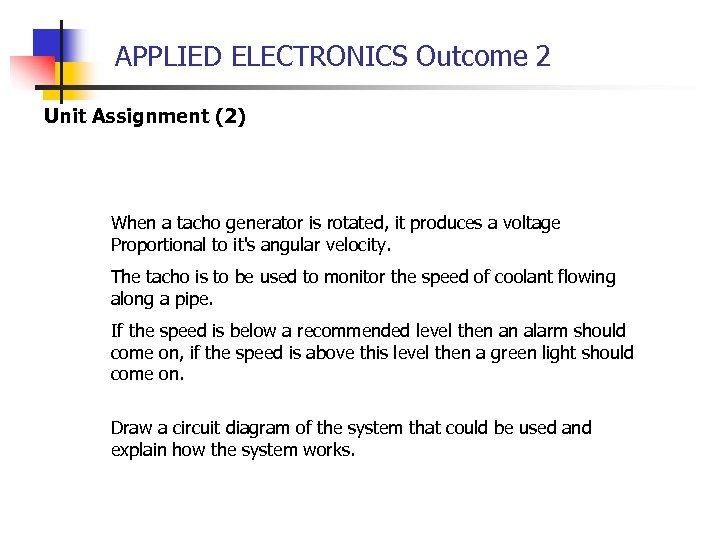APPLIED ELECTRONICS Outcome 2 Unit Assignment (2) When a tacho generator is rotated, it produces a voltage Proportional to it's angular velocity. The tacho is to be used to monitor the speed of coolant flowing along a pipe. If the speed is below a recommended level then an alarm should come on, if the speed is above this level then a green light should come on. Draw a circuit diagram of the system that could be used and explain how the system works.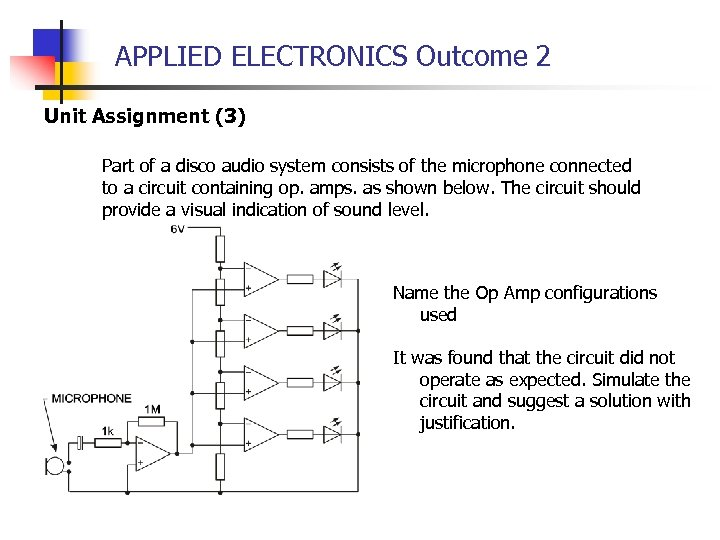APPLIED ELECTRONICS Outcome 2 Unit Assignment (3) Part of a disco audio system consists of the microphone connected to a circuit containing op. amps. as shown below. The circuit should provide a visual indication of sound level. Name the Op Amp configurations used It was found that the circuit did not operate as expected. Simulate the circuit and suggest a solution with justification.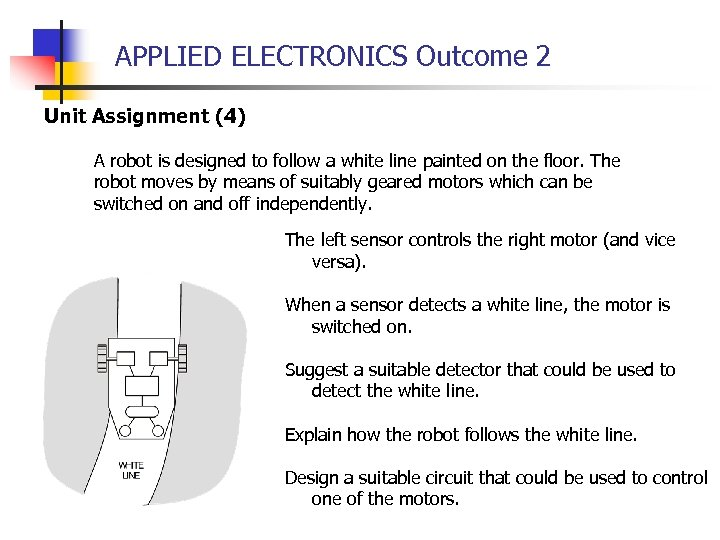APPLIED ELECTRONICS Outcome 2 Unit Assignment (4) A robot is designed to follow a white line painted on the floor. The robot moves by means of suitably geared motors which can be switched on and off independently. The left sensor controls the right motor (and vice versa). When a sensor detects a white line, the motor is switched on. Suggest a suitable detector that could be used to detect the white line. Explain how the robot follows the white line. Design a suitable circuit that could be used to control one of the motors.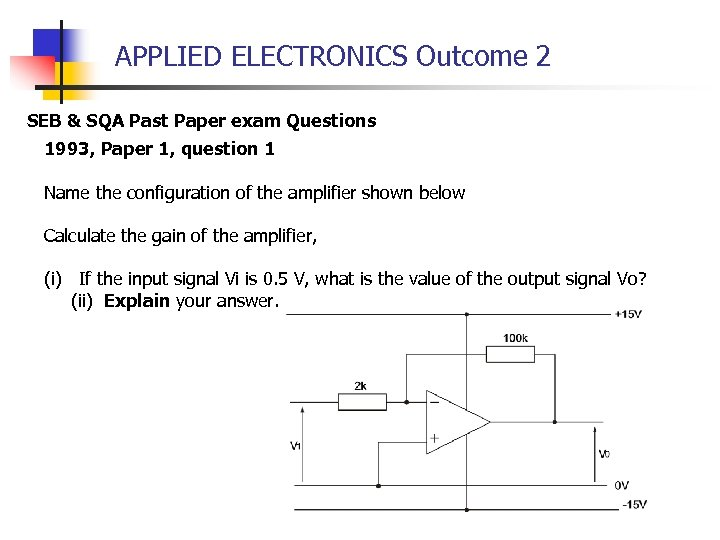APPLIED ELECTRONICS Outcome 2 SEB & SQA Past Paper exam Questions 1993, Paper 1, question 1 Name the configuration of the amplifier shown below Calculate the gain of the amplifier, (i) If the input signal Vi is 0. 5 V, what is the value of the output signal Vo? (ii) Explain your answer.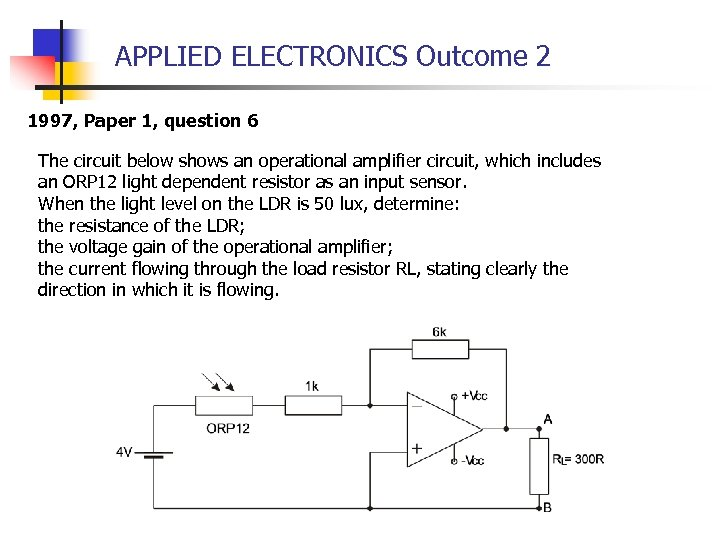APPLIED ELECTRONICS Outcome 2 1997, Paper 1, question 6 The circuit below shows an operational amplifier circuit, which includes an ORP 12 light dependent resistor as an input sensor. When the light level on the LDR is 50 lux, determine: the resistance of the LDR; the voltage gain of the operational amplifier; the current flowing through the load resistor RL, stating clearly the direction in which it is flowing.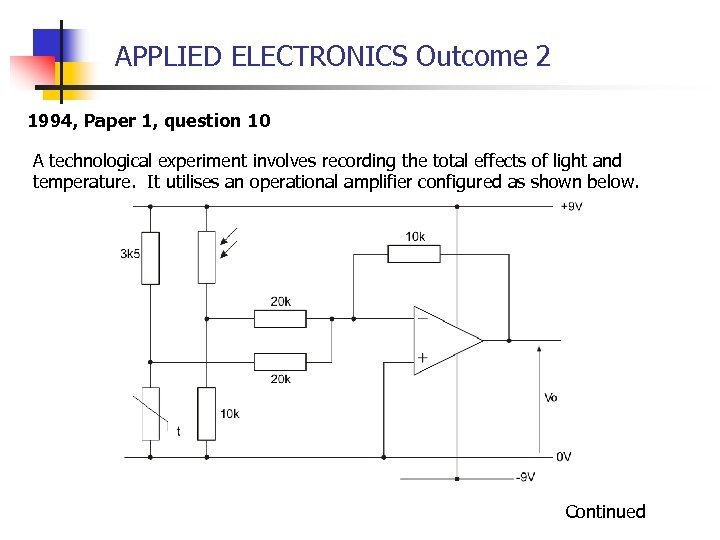APPLIED ELECTRONICS Outcome 2 1994, Paper 1, question 10 A technological experiment involves recording the total effects of light and temperature. It utilises an operational amplifier configured as shown below. Continued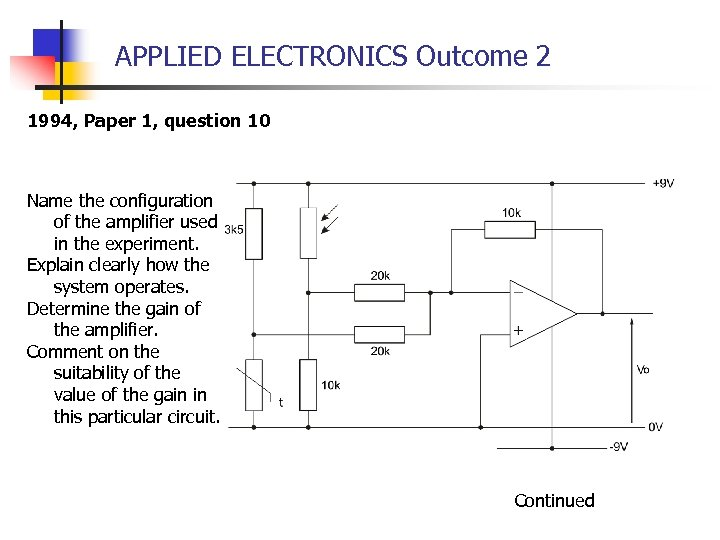APPLIED ELECTRONICS Outcome 2 1994, Paper 1, question 10 Name the configuration of the amplifier used in the experiment. Explain clearly how the system operates. Determine the gain of the amplifier. Comment on the suitability of the value of the gain in this particular circuit. Continued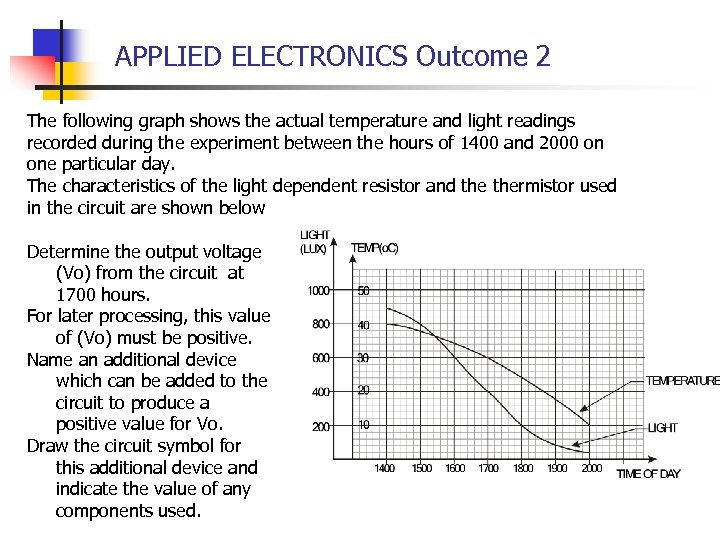APPLIED ELECTRONICS Outcome 2 The following graph shows the actual temperature and light readings recorded during the experiment between the hours of 1400 and 2000 on one particular day. The characteristics of the light dependent resistor and thermistor used in the circuit are shown below Determine the output voltage (Vo) from the circuit at 1700 hours. For later processing, this value of (Vo) must be positive. Name an additional device which can be added to the circuit to produce a positive value for Vo. Draw the circuit symbol for this additional device and indicate the value of any components used.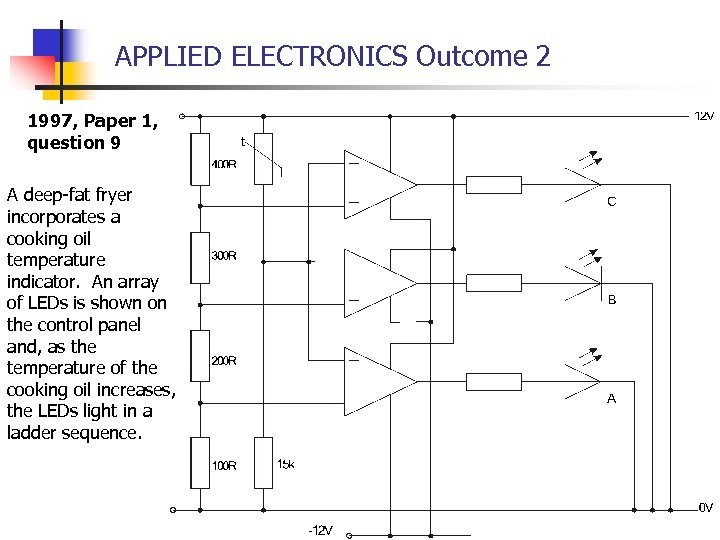APPLIED ELECTRONICS Outcome 2 1997, Paper 1, question 9 A deep-fat fryer incorporates a cooking oil temperature indicator. An array of LEDs is shown on the control panel and, as the temperature of the cooking oil increases, the LEDs light in a ladder sequence.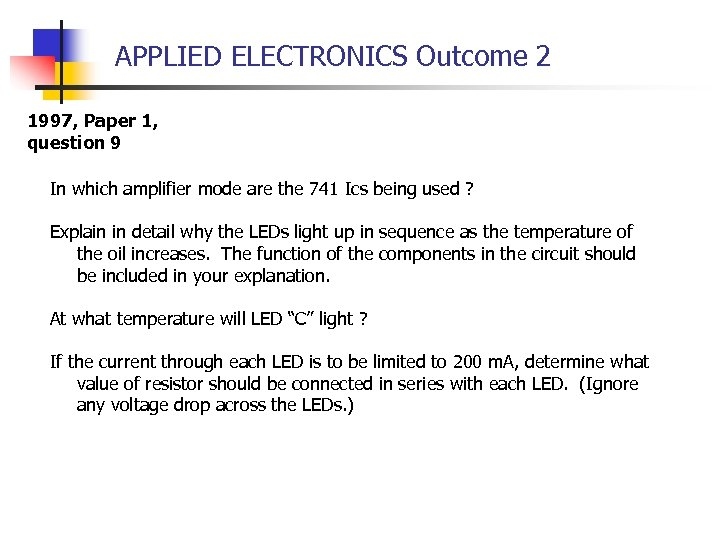APPLIED ELECTRONICS Outcome 2 1997, Paper 1, question 9 In which amplifier mode are the 741 Ics being used ? Explain in detail why the LEDs light up in sequence as the temperature of the oil increases. The function of the components in the circuit should be included in your explanation. At what temperature will LED “C” light ? If the current through each LED is to be limited to 200 m. A, determine what value of resistor should be connected in series with each LED. (Ignore any voltage drop across the LEDs. )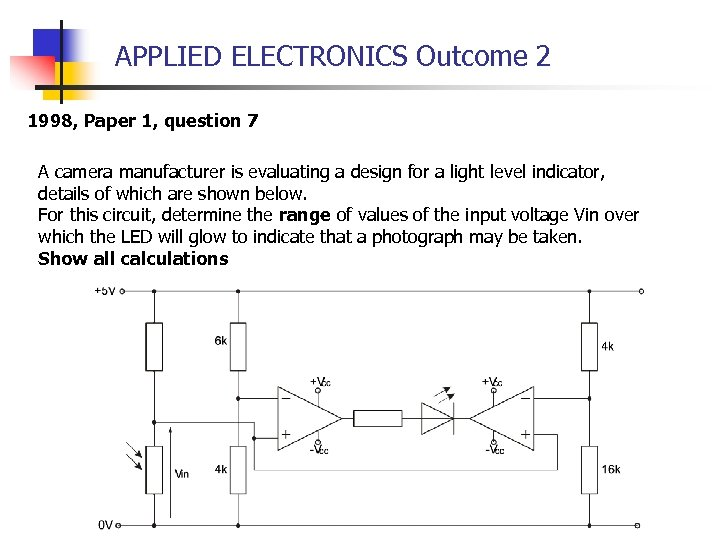APPLIED ELECTRONICS Outcome 2 1998, Paper 1, question 7 A camera manufacturer is evaluating a design for a light level indicator, details of which are shown below. For this circuit, determine the range of values of the input voltage Vin over which the LED will glow to indicate that a photograph may be taken. Show all calculations